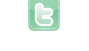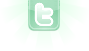# Shooter game

I'm not entirely sure if I'm correct but: - Unless it's declared else where, I don't think MoveUp, MoveDown/Left/Right are Greenfoot methods, instead you can try: • move(x) : if x is a positive number, it'll move forward, if x is negate, it'll move backward (according to its rotation) • setLocation( getX()+x, getY()+x) : similar to move(), but you can also go in the Y axis - Here's what that might look like: if(Greenfoot.isKeyDown("up")) setLocation(getX()+5, getY()-5); //make sure you have the () after getX and getY otherwise it won't work //if you're going up, a negative integer is needed to decrease the distance from the Bottom of the world if(Greenfoot.isKeyDown("right")) move(5); if(Greenfoot.isKeyDown("down")) setLocation(getX(), getY()+5); if(Greenfoot.isKeyDown("left")) move(-5); .... - Lastly, you might wanna check to see if you have the Vector Class with the correct information in your Scenario Hopefully this helps, but just know that I'm not a master programmer lol
What do you mean by "the code is not working in my favor"? That is vague. How is it not what you want? What is it doing that is should not do and what should it do that it is not doing?
to answer the first question I have a subclass of actor that is called mover and that is where I called all of the move()'s. here is the code as well because I am having some issues with the moving and the Y axis and X axis are not liking the double that I put next to it although I did an int at the top. public abstract class Mover extends Actor { int x=0; int y; public Vector movement = new Vector(); /** * Act - do whatever the Mover wants to do. This method is called whenever * the 'Act' or 'Run' button gets pressed in the environment. */ public void act() { } //public Mover(Vector speed) { //movement = speed; } public void moveRight() { // setLocation(getX() + zoom, getY()); } public void moveUp() { //setLocation(getX(), getY() + zoom); } public void moveDown() { //setLocation(getX(), getY()-zoom); } public void moveLeft() { //setLocation(getX() - zoom, getY()); } public void moveAll() { double x=x+movement.getX(); double y=y+movement.getY(); if(x>= getWorld().getWidth()) x=0; if(x<0) x=getWorld().getWidth()-1; if(y>=getWorld().getHeight()) y=0; if(y<0) y=getWorld().getHeight()-1; } }
Pie8756 wrote...
the Y axis and X axis are not liking the double that I put next to it
Again, this is vague. Is it underlining something? If so, what is being underlined and what error message do you get when hovering the mouse over it? Also, please provide the following: (1) Vector class codes; (2) your world constructor codes;
public class Vector { double dx = 0; double dy = 0; int direction = 0; double length; /** * Create a default vector initialised to zero. */ public Vector() { } /** * Create a vector with given direction and length. */ public Vector(int direction, double length) { this.length = length; this.direction = direction; dx = length * Math.cos(Math.toRadians(direction)); dy = length * Math.sin(Math.toRadians(direction)); } /** * Set the direction of this vector. */ public void setDirection(int direction) { this.direction = direction; dx = length * Math.cos(Math.toRadians(direction)); dy = length * Math.sin(Math.toRadians(direction)); } /** * Add another vector to this vector. */ public void add(Vector other) { dx += other.dx; dy += other.dy; this.direction = (int) Math.toDegrees(Math.atan2(dy, dx)); this.length = Math.sqrt(dx*dx+dy*dy); } /** * Return the x offset of this vector. */ public double getX() { return dx; } /** * Return the y offset of this vector. */ public double getY() { return dy; } /** * Return the current direction (in degrees). */ public int getDirection() { return direction; } /** * Return the current length of the vector. */ public double getLength() { return length; } /** * Create a copy of this vector. */ public Vector copy() { Vector copy = new Vector(); copy.dx = dx; copy.dy = dy; copy.direction = direction; copy.length = length; return copy; } } Here is my Vector class codes and here is the world code public World(int worldWidth, int worldHeight, int cellSize, boolean bounded) worldWidth - The width of the world (in cells). worldHeight - The height of the world (in cells). cellSize - Size of a cell in pixels. bounded - Should actors be restricted to the world boundary? I didn't really know exactly what you meant by that but I think that might be it. for the error messages that are showing up in Mover, it says variable y and Variable x might not have been initialized. So that is why I wrote the integers at the top. Also in flaco in private void fire() on .move() it says method move in class greenfoot.Actor cannot be applied to given types; required: int found: no arguments reason: actual and formal argument lists differ in length hopefully that is less vague
Pie8756 wrote...
here is the world code << World(int, int, boolean) API description Omitted >> I didn't really know exactly what you meant by that but I think that might be it.
for the error messages that are showing up in Mover, it says variable y and Variable x might not have been initialized. So that is why I wrote the integers at the top.
Remove first two lines in Mover class (int x=0; int y;). Use:
```double x = movement.getX();
double y = movement.getY();```
(you were using the declared variable inside the formula to initialize it)
Also in flaco in private void fire() on .move() it says method move in class greenfoot.Actor cannot be applied to given types; required: int found: no arguments reason: actual and formal argument lists differ in length
Need to see the Bullet class codes for this one.
Alright the code at the top that you gave me fixed the initial error but now the new code is saying that it is an illegal forward reference.
danpost wrote...
Pie8756 wrote...
here is the world code << World(int, int, boolean) API description Omitted >> I didn't really know exactly what you meant by that but I think that might be it.
No. Your code in your subclass of World where you create YOUR world. here is the Myworld code I haven't really put much into it. public class MyWorld extends World { /** * Constructor for objects of class MyWorld. * */ public MyWorld() { // Create a new world with 600x400 cells with a cell size of 1x1 pixels. super(600, 400, 1); } } And as well as the Bullet code public class Bullet extends Mover { /** A bullet looses one life each act, and will disappear when life = 0 */ private int life = 30; /** The damage this bullet will deal */ private int damage = 16; public Bullet() { } public Bullet(Vector speed, int rotation) { super(speed); setRotation(rotation); increaseSpeed(new Vector(rotation, 15)); Greenfoot.playSound("EnergyGun.wav"); } /** * The bullet will damage asteroids if it hits them. */ public void act() { if(life <= 0) { getWorld().removeObject(this); } else { move(); Asteroid asteroid = (Asteroid) getOneIntersectingObject(Asteroid.class); if (asteroid != null) { getWorld().removeObject(this); asteroid.hit(damage); } else { life--; } } } } the error that is showing up in Bullet is the Super(speed); it is saying the same error message as in Flaco and not that I look at it super is also in My world so it might have to do with something in there.
In the Mover class above, you have lines commented out that probably should not be. Make sure that you have the following in that class:
```public Mover() {}

public Mover(Vector speed)
{
movement = speed;
}```